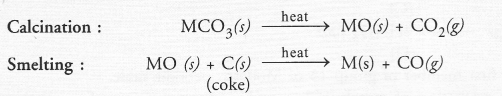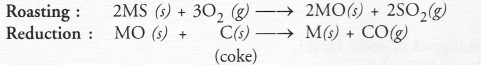## NCERT Exemplar Solutions for Class 10 Science Chapter 3 Metals and Non-metals

These Solutions are part of NCERT Exemplar Solutions for Class 10 Science. Here we have given NCERT Exemplar Solutions for Class 10 Science Chapter 3 Metals and Non-metals

NCERT Exemplar Solutions for Class 10 Science Chapter 3 Multiple Choice Questions

Question 1.
Which of the following properties is generally not shown by metals ?
(a) Electrical conduction
(b) Sonorous nature
(c) Dullness
(d) Ductility.
(c). Metals have shining lustre and they mostly do not show any dullness.

More Resources

Question 2.
The ability of metals to be drawn into thin sheets is known as
(a) ductility
(b) malleability
(c) sonorosity
(d) conductivity.
(b).

Question 3.
Aluminium is used for making cooking utensils. Which of the following properties of the metal are responsible for the same ?
(i) Good thermal conductivity
(ii) Good electrical conductivity
(iii) Ductility
(iv) High melting point
(a) (i) and (ii)
(b) (i) and (iii)
(c) (ii) and (iii)
(d) (i) and (iv)
(d). In order that a metal may be used for making cooking utensils, it must be good conductor of heat and also must have high melting point.

Question 4.
Which one of the following metals does not react with cold as well as hot water ?
(a) K
(b) Ca
(c) Mg
(d) Fe.
(d).

Question 5.
Which of the following oxide(s) of iron would be obtained on prolonged reaction of iron with steam ?
(a) FeO
(b) Fe2O3
(c) Fe3O4
(d) Fe2O3 and Fe3O4
(c).
3Fe (s) + 4H2O (g) ———> Fe3O4 (s) + 4H2 (g)

Question 6.
What happens when magnesium is treated with water ?
(i) It does not react with water
(ii) It reacts violently with water
(iii) It reacts less violently with water
(iv) Bubbles of hydrogen gas formed stick to the surface of the metal
(a) (i) and (iv)
(b) (ii) and (iii)
(c) (i) and (ii)
(d) (iii) and (iv)
(d).
(iii) Magnesium reacts with water slowly to form magnesium hydroxide along with hydrogen gas
Mg(s) + 2H2O(l) ———-> Mg(OH)2(s) + H2(g)
(iv) Bubbles of hydrogen gas stick to the surface of the metal.

Question 7.
Generally metals react with acids to give salt and hydrogen gas. Which of the following acids does not give hydrogen gas on reacting with metals (except Mn and Mg) ?
(a) H2SO4
(b) HCl
(c) HNO3
(d) All of these.
(c). HNO3 is a strong oxidising agent. It oxidises metals to metal oxides and then to metal nitrates and itself is reduced to either nitric oxide (NO), nitruos oxide (N2O) or nitrogen dioxide (NO2). However, both Mg and Mn evolve hydrogen on reacting with the acid.

Question 8.
Composition of aqua-regia by volume is :
(a) Dil HCl (3) : Cone HNO3 (1)
(b) Cone HCl (3) : Dil HNO3 (1)
(c) Cone HCl (3) : Cone HNO3 (1)
(d) Dil HC1 (3) : Dil HNO3 (1)

Question 9.
Which of the following are not ionic compounds ?
(i) CaCl2
(ii) HCl
(iii) CCl4
(iv) NaCl
(a) (i) and (ii)
(b) (ii) and (iii)
(c) (iii) and (iv)
(d) (i) and (iii)
(b). Both HCl and CCl4 are covalent compounds in nature.

Question 10.
Which one of the following properties is not generally exhibited by ionic compounds ?
(a) Solubility in water
(b) Electrical conductivity in solid state
(c) High melting and boiling points
(d) Electrical conductivity in molten state.
(b). Ionic compounds are conducting due to the movement of ions. Since the ions do not move in the solid state, these compounds are therefore, not conducting in the solid state.

Question 11.
Which of the following metals exist in their native state in nature ?
(i) Cu
(ii) Au
(iii) Zn
(iv) Ag
(a) (i) and (ii)
(b) (ii) and (iii)
(c) (ii) and (iv)
(d) (iii) and (iv)
(c). Both Au and Ag are very little reactive. They exist in native state. However, the metal silver may also exist in some combined states (as minerals).

Question 12.
Metals are refined by using different methods. Which of the following metals are refined by electrolytic refining ?
(i) Ag
(ii) Cu
(iii) Na
(iv) Mg
(a) (i) and (ii)
(b) (i) and (iii)
(c) (ii) and (iii)
(d) (iii) and (iv)
(a). Both Ag and Cu are very hard. These are called coinage metals and are purified by electrolytic refining.

Question 13.
Silver articles become black on prolonged exposure to air. This is due to the formation of
(a) AgCN
(b) Ag2O
(c) Ag2S
(d) Ag2S and AgCN
(c). Air contains traces of hydrogen sulphide (H2S) gas which reacts with silver (Ag) to form Ag2S black in colour.

Question 14.
Galvanisation is a method of protecting iron from rusting by coating with a thin layer of
(a) Chromium
(b) Copper
(c) Zinc
(d) Tin
(c). Coating the surface of iron with zinc is called galvanization.

Question 15.
Stainless steel is a very useful material for our life. In stainless steel, iron is mixed with
(a) Ni and Cr
(b) Cu and Cr
(c) Ni and Cu
(d) Cu and Au.

Question 16.
If copper is kept open in air, it slowly loses its shining brown surface and gains a green coating. It is due to the formation of :
(a) CuSO4
(b) CUCO3CO(OH)2
(c) CU(NO3)2
(d) CuO.
(b). The green coating is due to the formation of basic copper carbonate which is CuCO3.Cu(OH)2.

Question 17.
Generally, metals are solid in nature. Which one of the following metals is found in liquid state at room temperature ?
(a) Na
(b) Fe
(c) Al
(d) Hg
(d). Mercury (Hg) is a liquid at room temperature.

Question 18.
Which of the following metals are obtained by electrolysis of their chlorides in molten state ?
(i) Na
(ii) Ca
(iii) Fe
(iv) Cu
(a) (i) and (iv)
(b) (iii) and (iv)
(c) (i) and (iii)
(d) (i) and (ii)
(d). Both Na and Ca are obtained by the electrolysis of their molten chlorides.

Question 19.
Generally non-metals are not lustrous. Which of the following non-metals is lustrous ?
(a) Sulphur
(b) Phosphorus
(c) Nitrogen
(d) Iodine.
(d). Iodine is lustrous.

Question 20.
Which one of the following four metals would be displaced from the solution of its salts by other three metals ?
(a) Mg
(b) Cu
(c) Zn
(d) Fe
(b). Cu is the least reactive and would be displaced from its salts by other metals.

Question 21.
5 mL each of concentrated HCl, HNO3 and a mixture of concentrated HCl (15 mL) and concentrated HNO3 (5 mL) were taken in test tubes labelled as A, B and C. A small piece of metal was put in each test tube. No change occurred in test tubes A and B but the metal got dissolved in test tube C. The metal could be
(a) Al
(b) Au
(c) Cu
(d) Ag
(b). Gold (Au) dissolves in aqua regia.

Question 22.
An alloy is :
(a) an element
(b) a compound
(c) a homogeneous mixture
(d) a heterogeneous mixture
(c). An alloy is a homogeneous mixture of two or more elements (metals or non-metals.)

Question 23.
An electrolytic cell consists of :
(i) positively charged cathode
(ii) negatively charged anode
(iii) positively charged anode
(iv) negatively charged cathode
(a) (i) and (ii)
(b) (iii) and (iv)
(c) (i) and (iii)
(d) (ii) and (iv)
(b).

Question 24.
During electrolytic refining of copper, ir gets
(a) deposited on cathode
(b) deposited on anode
(c) deposited on cathode as well as anode
(d) remains in the solution.
(a). Pure metal is always deposited on cathode.

Question 25.
An element ‘X’ is soft and can be cut with a knife. This is very reactive to air and cannot be kept in open air. It reacts vigorously with water. Identify the element from the following
(a) Mg
(b) Na
(c) S
(d) Mg
(b). Sodium (Na) gives all the characteristics of the element X.

Question 26.
Alloys are homogeneous mixtures of a metal with a metal or non-metal. Which among the following alloys contain non-metal as one of its constituents ?
(a) Brass
(b) Gun metal
(c) Amalgam
(d) Steel.
(d). Steel (stainless) contains very small percentage of carbon.

Question 27.
Which of the following statements is not correct for magnesium metal ?
(a) It burns in oxygen with dazzling flame
(b) It reacts with cold water to form magnesium oxide and evolves hydrogen gas
(c) It reacts with hot water to form magnesium hydroxide and evolves hydrogen gas
(d) It reacts with steam to form magnesium hydroxide and evolves hydrogen gas.
(b). Magnesium (Mg) does not react with cold water since its position in the reactivity series of metals is not at the top.

Question 28.
Which among the following alloys contain mercury as one of its constituents ?
(a) Stainless steel
(b) German silver
(c) Solder
(d) Zinc amalgam.
(d). All amalgams contain mercury as one of their constituents.

Question 29.
Reaction between the elements X and Y results in the compound Z. Whereas X loses electron, Y gains the same. Which of the following properties is not shown by Z ?
(a) Has high melting point
(b) Has low melting point
(c) Conducts electricity in molten state
(d) Occurs as solid
(b). Z is an ionic compound. Therefore, it is not expected to have low melting point.

Question 30.
The electronic configurations of three elements X, Y and Z are X — 2, 8; Y — 2, 8, 6 and Z — 2, 8, 1. Which of the following is correct ?
(a) X is a metal
(b) Y is a metal
(c) Z is a non-metal
(d) Y is a non-metal and Z is a metal.
(d). Y is sulphur (S) while Z is sodium (Na)

Question 31.
Although metals form basic oxides, which of the following metals form an amphoteric oxide ?
(a) Na
(b) Ca
(c) Zn
(d) Cu.
(c). Zn forms amphoteric oxide ZnO which reacts with both the acids and the alkalies.

Question 32.
Generally, non-metals are not conductors of electricity. Which of the following is a good conductor of electricity ?
(a) Diamond
(b) Graphite
(c) Phosphorus
(d) Iodine
(b). Graphite is a good conductor of electricity.

Question 33.
Electrical wires have a coating of an insulating material. The material, generally used is
(b) Graphite
(c) PVC
(d) All can be used.
(c). PVC (Poly Vinyl Chloride) coatings are generally used for insulating electrical wires.

Question 34.
Which of the following non-metals is a liquid ?
(a) Carbon
(b) Bromine
(c) Iodine
(d) Sulphur
(b). Non-metal bromine is a liquid but in the molecular form (Br2).

Question 35.
Which of the following can undergo a chemical reaction ?
(a) MgSO4 + Zn
(b) ZnSO4 + Fe
(c) CaSO4 + Pb
(d) CuSO4 + Al
(d). Only Al can displace Cu from CuSO4 solution since it is placed above Cu in the activity series.

Question 36.
Which one of the following figures correctly describes the process of electrolytic refining ?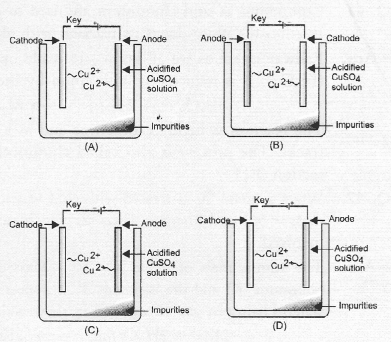(c). Cu2+ ions are released from anode by the oxidation of copper (Cu). These migrate towards cathode and are reduced to copper (Cu).

NCERT Exemplar Solutions for Class 10 Science Chapter 3 Short Answer Questions

Question 37.
Iqbal treated a lustrous, divalent element M with sodium hydroxide. He observed the formation of bubbles in reaction mixture. He made the same observations when this element was treated with hydrochloric acid. Suggest how can he identify the produced gas. Write chemical equations for both the reactions.
The divalent element M is a metal. It reacts with both sodium hydroxide (alkali) and dilute hydrochloric acid to evolve hydrogen gas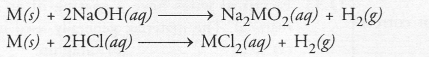The gas burns with a pop sound when a burning match stick is brought near it.

Question 38.
During extraction of metals, electrolytic refining is used to obtain pure metals,
(a) Which material will be used as anode and cathode for refining of copper metal by this process
(b) Suggest a suitable electrolyte also,
(c) In this electrolytic cell, where do we get pure copper after passing electric current ?
In the electrolytic refining of impure copper metal :
(a) A rod of impure copper is used as anode while that of pure copper as cathode.
(b) The electrolyte is a water soluble salt of copper. It is normally an aqueous solution of copper sulphate.
(c) On passing current, pure copper gets deposited at the cathode. It can be scrapped off later on. For more details.

Question 39.
Why should the metal sulphides and carbonates be converted to metal oxides in the process of extraction of metal from them ?
It is quite easy to obtain a metal from metal oxide by carrying its reduction with a suitable reducing agent. However, metal sulphides and carbonates are converted to the oxide form and then reduced.

Question 40.
Generally, when metals are treated with mineral acids, hydrogen gas is liberated but when metals (except Mn and Mg), are treated with HNCfy, hydrogen is not liberated, why ?
HNO3 acts as a powerful oxidising agent along with acting as acid. In this case, hydrogen gas which is intially involved is oxidised to water.

Question 41.
Compound A’ and aluminium are used to join railway tracks,
(a) Identify the compound A’
(b) Name the reaction
(c) Write down its reaction with aluminium.
Railway trakcs as we all know, are made up of iron. This means that the compound A is an oxide of iron (Fe2CO3). It is reduced by aluminium by thermit reaction with aluminium.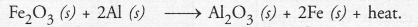The reaction is highly exothermic and as a result, iron is in molten state and can weld the broken railway tracks. The process is also called aluminothermie reduction or thermit process.

Question 42.
When a metal ‘X’ is treated with cold water, it gives a basic salt ‘Y’ with molecular formula XOH (Molecular mass = 56) and liberates a gas ‘Z’ which easily catches fire. Identify X, Y and Z and also write the reaction involved.
The atomic mass of metal X = 56 – Mass of OH group = 56 — 17 = 39u. This shows that the metal ‘X’ is potassium (K) and the basic salt ‘Y’ is potassium hydroxide (KOH). It is formed by reacting potassium with cold water. Hydrogen gas ‘Z’ evolved in the reaction catches fire.Question 43.
A non-metal X-exists in two different forms Y and Z. Y is the hardest natural substance, whereas Z is a good conductor of electricity. Identify X, Y and Z.
Since “Y” is the hardest occuring non-metal, it is diamond. The non-metal “X” is carbon. Y is the crystalline allotropie form of carbon. The other allotropie form Z which is a good conductor of electricity is graphite.

Question 44.
The following reaction takes place when aluminium powder is heated with Mn02.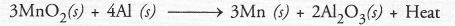(a) Is aluminium getting reduced ?
(b) Is Mn02 getting oxidised ?
(a) Aluminium is getting oxidised to Al2O3.
(b) Manganese dioxide is getting reduced to Mn.

Question 45.
What are the constituents of solder alloy ? Which property of solder makes it suitable for welding electrical wires ?
The constituents of solder alloy are ; lead (Pb) and tin (Sn). Being a low melting solid and also a good conductor of electricity, solder is used for welding electrical wires and cables.

Question 46.
A metal ‘M’, which is used in thermite process, when heated with oxygen gives an oxide, which is amphoteric in nature. Identify ‘M’ and its oxide. Write down the reactions of oxide with HCl and NaOH.
The information suggests that the metal ‘M’ is aluminium (Al). The formula of its oxide is Al2O3 which is amphoteric in nature. It reacts with both acid and base.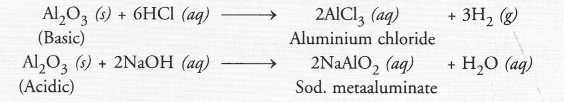Question 47.
A metal that exists as a liquid at room temperature is obtained by heating its sulphide in the presence of air. Identify the metal and its ore and give the reaction involved.
Mercury is the metal which exists as a liquid at room temper nature. It can be obtained by heating the sulphide ore in the presence of air. The process is known as roasting. The chemical reactions involved are :Question 48.
Give the formulae of the stable binary compounds that would be formed by the combination of following pairs of elements.
(a) Ca and N2
(b) Li and O2
(c) Ca and Cl2
(d) K and
(a) Calcium nitride (Ca3N2)
(b) Lithium oxide (Li2O)
(c) Calcium chloride (CaCl2)
(d) Potassium oxide (K2O).

Question 49.
What happens when
(a) ZnCO3 is heated in the absence of oxygen ?
(b) a mixture of Cu2O and Cu2S is heated ?
(a) A mixture of ZnO(s) and CO2 is formed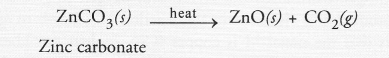(b) A mixture of copper and SO2 is formed.Question 50.
A non-metal A is an important constituent of our food and forms two oxides B and C. Oxide B is toxic whereas C causes global warming
(a) Identify A, B and C
(b) To which Group of Periodic Table does A belong ?
The non-metal A is carbon. It is an important constituent of our food in different forms. For example, glucose (C6H12O6) contains carbon. In fact, all food materials are organic compounds and these contain carbon as essential constituent. The two oxides of carbon are, carbon monoxide (B) and carbon dioxide (C). Carbon dioxide causes global warming.
(a) A = Carbon (C) ;
B = Carbon monoxide (CO) ;
C = Carbon dioxide (CO2)
(b) Carbon is the first member of group 14 in the Long form of Periodic Table.

Question 51.
Give two examples each of the metals that are good conductors and poor conductors of heat respectively.
Good conductors of heat : Ag and Cu Poor conductors of heat : Pb and Hg.

Question 52.
Name one metal and one non-metal that exist in liquid state at room temperature. Also name two metals having melting point less than 310 K (37°C).
The metal mercury (Hg) and non-metal (Br2) exist in liquid state at room temperature.
The two metals with melting points less than 310 K are ; cesium (Cs) with melting point 301 K and gallium (Ga) with melting point 303 K.

Question 53.
An element ‘A’ reacts with water to form a compound ‘B’ which is used in white washing. The compound ‘B’ on heating forms an oxide ‘C’ which on treatment with water gives back ‘B’. Identify ‘A’, ‘B’ and ‘C’ and give the reactions involved.
Since the compound ‘B’ is used for white wash, it is calcium hydroxide and the element ‘A’ is calcium. Upon heating ‘B’ forms calcium oxide ‘C’. It reacts with water to give calcium hydroxide ‘B’ again.Question 54.
An alkali metal A gives a compound B (molecular mass = 56) on reacting with water. The compound ‘B’ gives a soluble compound ‘C’ on treatment with aluminium oxide. Identify A, B and C and give the reaction involved.
As explained under Question 42, the alkali metal A is potassium (K) and on reacting with water, it forms a compound ‘B’ which is potassium hydroxide. This upon reacting with aluminium oxide (Al2O3) forms potassium metaluminate ‘C’.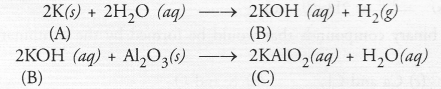Question 55.
Give the reaction involved during extraction of zinc from its ore by
(a) roasting of zinc ore
(b) calcination of zinc oreQuestion 56.
A metal M does not liberate hydrogen from acids but reacts with oxygen to give a black coloured product. Identify M and black coloured product and also explain the reaction of M with oxygen.
The avilable information suggests that the metal M is copper (Cu). It does not liberate hydrogen since it is placed below hydrogen in the reactivity series. Copper reacts with oxygen upon heating to form cupric oxide (CuO) which is black in colour.Question 57.
An element forms an oxide A2O3 which is acidic in nature. Identify A as a metal or non-metal.
Since the oxide A2O3 is acidic in nature, the element A is non-metal.

Question 58.
A solution of CuSO4 was kept in an iron pot. After few days, the iron pot was found to have a number of holes in it. Explain the reason in terms of reactivity. Write the equation of the reaction involved.
Iron (Fe) is placed above copper (Cu) in the reactivity series. This means that a chemical reaction had occurred between iron (iron pot) and aqueous CuSO4 solution.Since iron was consumed in the reaction, a number of holes appeared in the pot.

NCERT Exemplar Solutions for Class 10 Science Chapter 3 Long Answer Questions

Question 59.
A non-metal A which is the largest constituent of air, when heated with H2 in 1 : 3 ratio in the presence of catalyst (Fe) gives a gas B. On heating with O2 it gives an oxide C. If this oxide is passed into water in the presence of air, it gives an acid D which acts as a strong oxidising agent.
(a) Identify A, B, C and D.
(b) To which group of periodic table does this non-metal belong ?
(a) The available information suggests that the non-metal A is nitrogen (N) and its molecular form is N2. It is the major constituent of air (about 79 per cent by volume). It reacts with H2 upon heating m the presence of iron catalyst (Fe) to form ammonia (NH3) gas(g)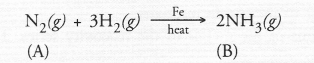Upon heating with oxygen, nitrogen forms intially nitric oxide (NO) and then nitrogen dioxide (C). The latter reacts with water in the presence of oxygen to form nitric acid (D). The acid acts as strong oxidising agent.(b) The element nitrogen is the first member of group 15 of Modern periodic table.

Question 60.
Give the steps involved in the extraction of metals of low and medium reactivity from their respective sulphide ores.
Extraction of Metals present low in the Activity series
Silver (Ag), gold (Au) and platinum (Pt) generally occur in the free or native state. This means that they can be isolated rather easily. Metals like copper (Cu) and mercury (Hg) are comparitively more reactive and occur in combined states. For example, the ore of mercury is cinnabar (HgS) while that of copper is copper glance (Cu2S). Both are converted into metallic form upon heating in air or oxygen.Extraction of Metals present in the middle of the Activity series
The metals present in the middle of the series are zinc, iron, chromium, nickel, cobalt, lead etc. These are usually present as sulphides or carbonares in nature. However, it is quite easy to obtain a metal from its oxide form which is then reduced to the metallic state. The various steps involved in the process of extraction are briefly discussed.
(a) Calcination :
Calcination may be defined as the process of heating the ore below its melting point in the absence of air.
As a result of calcination, the following changes take place

• Moisture is driven out and the ore becomes dry.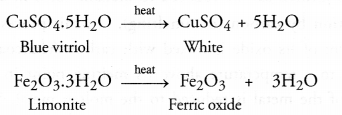• Some hydrated ores decompose and become anhydrous by losing molecules of water of crystallisation. For example, heat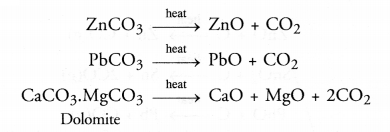(b) Roasting :
Roasting may be defined as the process of heating the ore below its melting point with excess of air.
As a result of roasting, the following changes occur :

• Any organic matter if present, gets destroyed.
• Impurities of non-metals such as sulphur, arsenic or phosphorus are converted into their volatile oxides which are removed. For example,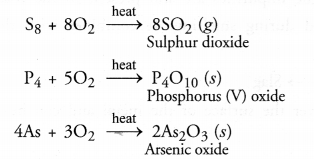• Sulphides of the metals are converted into their oxides. For example,• Small amounts of sulphides may also be converted into sulphates as as result of roasting. For example,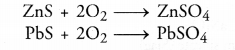Question 61.
Explain the following
(a) Reactivity of Al decreases if it is dipped in cone. HNO3
(b) Carbon cannot reduce the oxides of Na or Mg.
(c) NaCl is not a conductor of electricity in solid state whereas it does conduct electricity in aqueous solution as in molten state
(d) Iron articles are galvanised.
(e) Metals like Na, K, Ca and Mg are never found in their free state in nature.
(a) When Al metal is dipped in cone. HNO3 for sometime, it is oxidised initially to aluminium oxide (Al2O3). The oxide gets deposited on the surface of the metal and forms a protective coating an the surface. The metal is said to become passive towards air, acids and alkalies. Its reactivity therefore, decreases.
(b) Both Na and Mg are more reactive than carbon. Therefore, carbon is not in a position to reduce the oxides of these metals.
(c) NaCl is an ionic compound. Its electrical conductivity is due to the mobility of Na+ and Cl ions. These ions cannot move in the solid state. However, they can do so either in molten state of the salt or when it forms and aqueous solution in water.
(d) Iron has a tendency to get rusted in atmosphere by reacting with oxygen and water vapours present in air. In order to check rusting, iron articles are generally coated with zinc. This process is known as galvanization.
(e) All these metals are placed high in reactivity series of metals. They are quite reactive and do not exist in free states.

Question 62.
(i) Given below are the steps lor extraction of copper from its ore. Write the reaction involved.
(a) Roasting of copper (1) sulphide
(b) Reduction of copper (1) oxide with copper (1) sulphide.
(c) Electrolytic refining
(ii) Draw a neat and well labelled diagram for electrolytic refining of copper.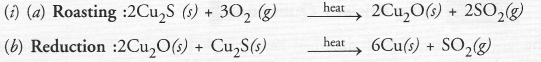This reaction in which one of the reactants (Cu2S) carries the reduction of the product (Cu2O) is known as auto-reduction.
(c) Reactions taking place in electrorefensing are :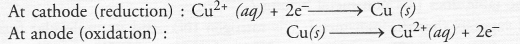(ii) For the diagram of electro-refining,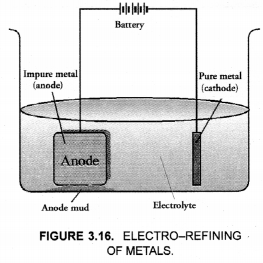Question 63.
Of the three metals X, Y and Z, X reacts with cold water, Y with hot water and Z with steam only. Identify X, Y and Z and also arrange them in order of increasing reactivity.
The answer is based on the relative positions of the metals in the reactivity series. The reactivity with water decreases down the series.

• Metal X is Na or K
• Metal Y is Mg or Ca
• Metal Z is Al or Fe.

Order of increasing reactivity : Z < Y < X.

Question 64.
Two ores ‘X’ and ‘Y’ were taken. On heating, ore ‘X’ gives CO2 whereas ore ‘Y’ gives SO2. What steps will you take to convert them into respective metals ?